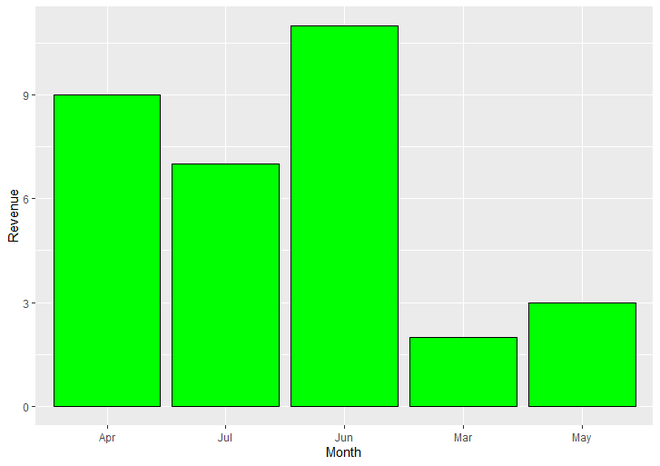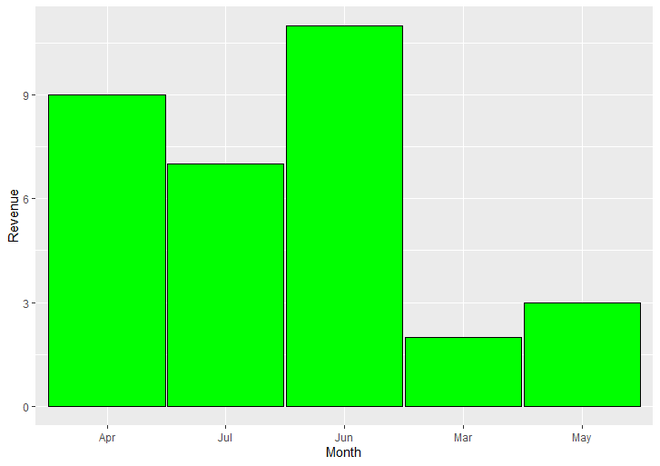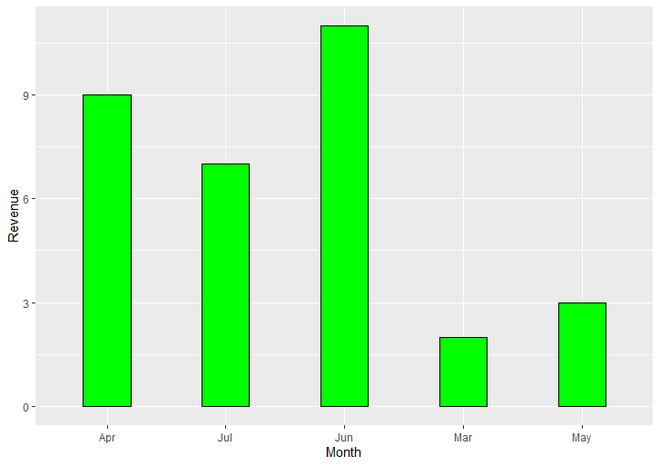# Change Space and Width of Bars in ggplot2 Barplot in R

• Last Updated : 23 May, 2021

In this article, we will see how to change the Space and width of bars in ggplot2 barplot in R.

For Create a simple Barplot using ggplot2, first we have to load the ggplot2 package using the library() function. If you have not already installed then you can install it by writing the below command in your R Console.

`install.packages("ggplot2")`

Then we create a DataFrame for plotting. In DataFrame, we use two vectors Month & Revenue. Named this dataframe as DF. Then we assign this DataFrame and vectors to a plot using ggplot() function. We set the Month vector to X-axis and Revenue to Y-axis. Then we make this plot Barplot by adding geom_bar() function to ggplot() function. inside geom_bar() function we set stat parameter to identity mode. By Default geom_bar uses stat=”bin”. This makes the height of each bar equal to the number of cases in each group. If we want the heights of bars to represent values in the data, we have to use stat=”identity” to map a value to the Y aesthetic. Here fill and color parameters are not necessary to use for creating BarPlot.

## R

 `# Load Package``library``(``"ggplot2"``)`` ` `# Create a DataFrame``DF <- ``data.frame``(Month = ``c``(``"Mar"``, ``"Apr"``, ``"May"``, ``"Jun"``, ``"Jul"``),    ``                   ``Revenue = ``c``(2, 9, 3, 11, 7))`` ` `# Create a Simple BarPlot using ggplot2``ggplot``(DF, ``aes``(Month, Revenue)) +               ``  ``geom_bar``(stat = ``"identity"``, fill = ``"green"``, color = ``"black"``)`

Output:BarPlot using ggplot2 in R

To Increase or Decrease width of Bars of BarPlot, we simply assign one more width parameter to geom_bar() function. We can give values from 0.00 to 1.00 as per our requirements. There will be two aspects that either we want to increase the width of bars and decrease space between bars, or we want to decrease the width of bars and increase space between bars.

Syntax : geom_bar(stat, fill, color, width)

Parameters :

• stat : Here we have set stat parameter to identity mode. Which is used when we want to the heights of bars to represent values in the data (Y aesthetic).
• fill : Represents Color of bars.
• color : Represents Color of border of the bars.
• width : Represents Width of the bars.

Return : ggplot2 BarPlot.

Example 1: Increase Width and Decrease Space between Bars

When we want to Increase the Width of Bars and Decrease Space between Bars, we simply have to use width parameter to the geom_bar() function. Here we set the value of the width parameter as 0.98. You can choose the value of ‘width’ parameter between 0.00 to 1.00 as per your requirements. When width of bars is increased, the Space between bars is automatically decreased. All other things are there as it was.

geom_bar(stat = “identity”, fill = “green”, color = “black”, width = 0.98)

Code:

## R

 `# Load Package``library``(``"ggplot2"``)`` ` `# Create DataFrame``DF <- ``data.frame``(Month = ``c``(``"Mar"``, ``"Apr"``, ``"May"``, ``"Jun"``, ``"Jul"``),    ``                   ``Revenue = ``c``(2, 9, 3, 11, 7))`` ` `# Create a BarPlot with increased width``# of Bars and decreased Space between Bars``ggplot``(DF, ``aes``(Month, Revenue))+               ``  ``geom_bar``(stat = ``"identity"``, fill = ``"green"``,``           ``color = ``"black"``, width = 0.98)`

Output:BarPlot with Increased width of Bars and Decreased Space between Bars

Example 2: Decrease Width and Increase Space between Bars.

When we want to Decrease the Width of Bars and Increase Space between Bars, we simply have to use the width parameter to the geom_bar() function. Here we set the value of the width parameter as 0.40. You can choose the value of ‘width’ parameter between 0.00 to 1.00 as per your requirements. When the width of bars is Decreased, the Space between bars is automatically increased. All other things are there as it was.

geom_bar(stat = “identity”, fill = “green”, color = “black”, width = 0.40)

Code:

## R

 `# Load Package``library``(``"ggplot2"``)`` ` `# Create DataFrame``DF <- ``data.frame``(Month = ``c``(``"Mar"``, ``"Apr"``, ``"May"``, ``"Jun"``, ``"Jul"``),    ``                   ``Revenue = ``c``(2, 9, 3, 11, 7))`` ` `# Create a BarPlot with decreased width of``# Bars and increased Space between Bars``ggplot``(DF, ``aes``(Month, Revenue))+               ``  ``geom_bar``(stat = ``"identity"``, fill = ``"green"``, ``           ``color = ``"black"``, width = 0.40)`

Output:BarPlot with Decreased width of Bars and Increased Space between Bars

My Personal Notes arrow_drop_up